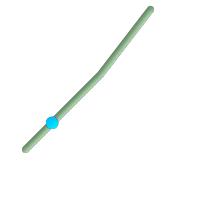## Name

ST_LineInterpolatePoint — Returns a point interpolated along a line. Second argument is a float8 between 0 and 1 representing fraction of total length of linestring the point has to be located.

## Synopsis

`geometry ST_LineInterpolatePoint(`geometry a_linestring, float8 a_fraction`)`;

## Description

Returns a point interpolated along a line. First argument must be a LINESTRING. Second argument is a float8 between 0 and 1 representing fraction of total linestring length the point has to be located.

See ST_LineLocatePoint for computing the line location nearest to a Point.Since release 1.1.1 this function also interpolates M and Z values (when present), while prior releases set them to 0.0.

Availability: 0.8.2, Z and M supported added in 1.1.1

Changed: 2.1.0. Up to 2.0.x this was called ST_Line_Interpolate_Point.This function supports 3d and will not drop the z-index.

## ExamplesA linestring with the interpolated point at 20% position (0.20)

```--Return point 20% along 2d line
SELECT ST_AsEWKT(ST_LineInterpolatePoint(the_line, 0.20))
FROM (SELECT ST_GeomFromEWKT('LINESTRING(25 50, 100 125, 150 190)') as the_line) As foo;
st_asewkt
----------------
POINT(51.5974135047432 76.5974135047432)

```
```--Return point mid-way of 3d line
SELECT ST_AsEWKT(ST_LineInterpolatePoint(the_line, 0.5))
FROM (SELECT ST_GeomFromEWKT('LINESTRING(1 2 3, 4 5 6, 6 7 8)') as the_line) As foo;

st_asewkt
--------------------
POINT(3.5 4.5 5.5)

--find closest point on a line to a point or other geometry
SELECT ST_AsText(ST_LineInterpolatePoint(foo.the_line, ST_LineLocatePoint(foo.the_line, ST_GeomFromText('POINT(4 3)'))))
FROM (SELECT ST_GeomFromText('LINESTRING(1 2, 4 5, 6 7)') As the_line) As foo;
st_astext
----------------
POINT(3 4)

```